### What Is FIFO in Rockwell Programming?

What Is FIFO in Rockwell Programming?

FIFO is a special programming instruction that can be used in the applications that require to store the sequence of data in order and unload it.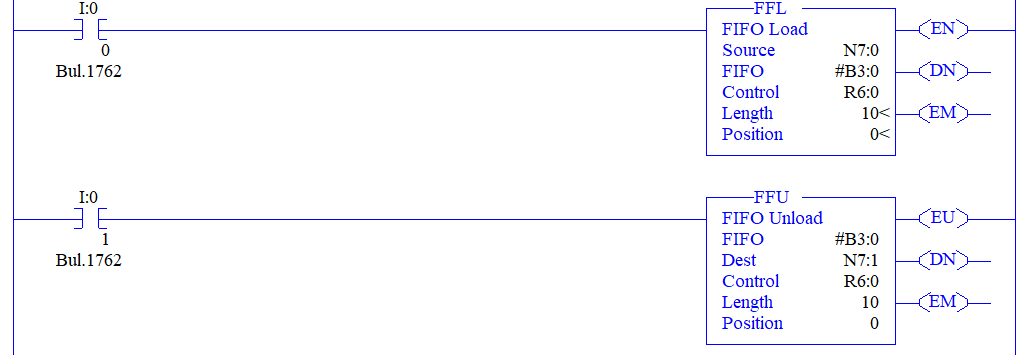Yes! FIFO should be used in a pair. There should be an instruction to load and there should be an instruction to unload.

The following are the block parameters in FIFO load instruction,

• Source - Here is where one should give an address where the values to be loaded are present.

• FIFO - Here is where one should specify the address where the values have to be loaded. This address seems weird right with an ‘#’ in the front. When we use an ‘#’ symbol in the front it is called an array of addresses. Obviously there will be a sequence of values present to be loaded arrays are capable of storing many values right? So why we gave ‘#’ in the front should make sense now.

• Control - Here is where one should specify the control address. In Rockwell PLC programming environment there is a separate address for the control with R6:0 as starting one. So when the FIFO load is done loading the values the R6:0 address gives us a true bit.

• Length: Here is where we have to specify the length of the array so that the array of address prepares them for loading the values.

• Position: Here is where we have to give the index number of an array from where the loading has to start. I’ll leave it to 0  so the loading starts from the 0th index.

The following are the block parameters in FIFO unload instruction,

• FIFO - Here is where one has to give the address where the values to be unloaded are present. So obviously both the load and unload has the same address in here.

• Dest - Here is where one has to give the address where the values have to be unloaded.

• Control, Length, and Position carry the same explanation from the FIFO load.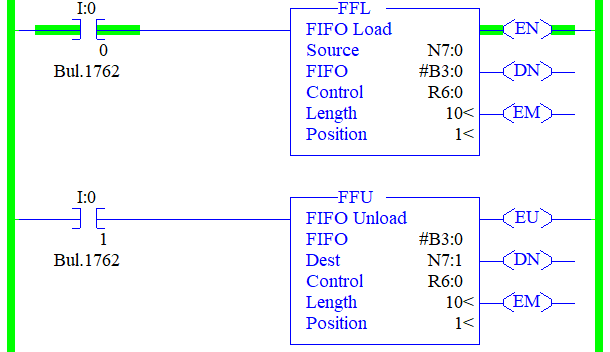Now we are on the run time and when the input to FFL is made to turn on the value on N7:0 gets loaded to the array address #B3:0. B3:0 is a binary address to view the value as decimal change the radix to decimal. Refer to the below figure for the first loaded value.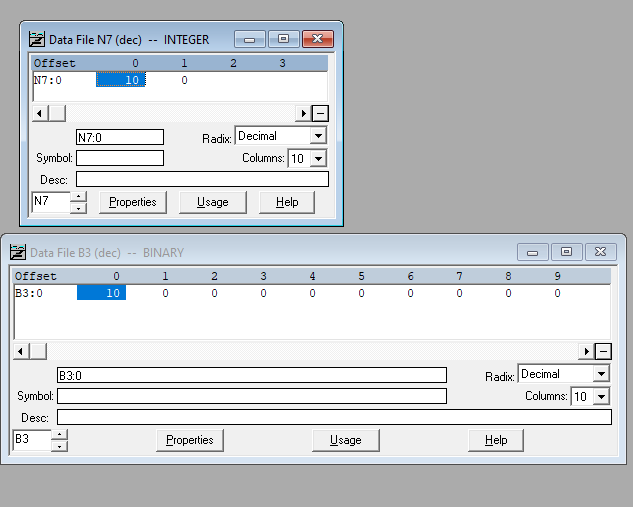You can also see the number of lengths we specified is 10 and the Binary data file shows us from 0 to 9.

Here is the next loaded value.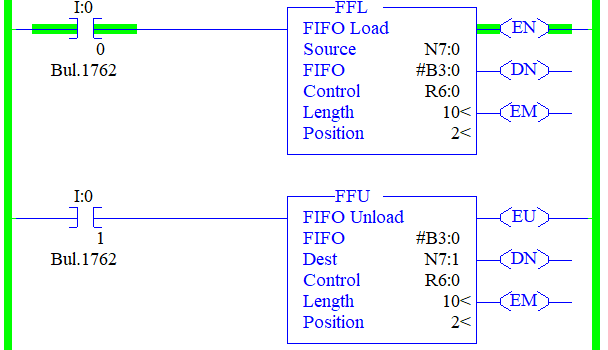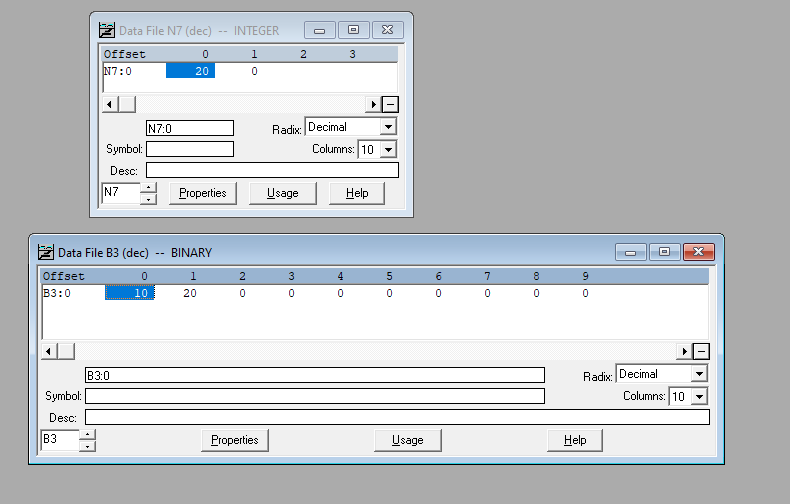You can see that the position is increasing in the FFL and FFU as the value is getting loaded. So the value is getting loaded from left to right the starting position is 0 and the ending is 9.

Also, notice that the value gets loaded for each false to the true transition of the rung. Refer to the below figure.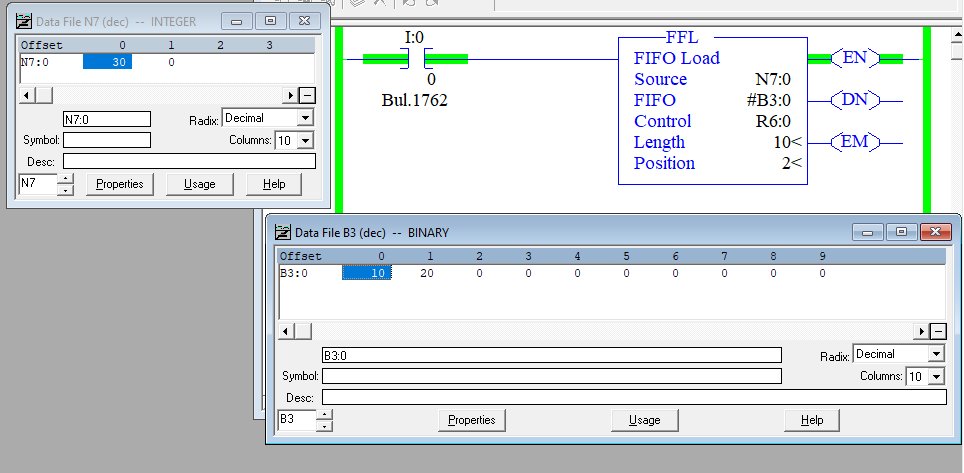You can see that I have changed the source address value to 30 but it is not getting loaded since the rung is staying true once the rung becomes false and true again then the value gets loaded.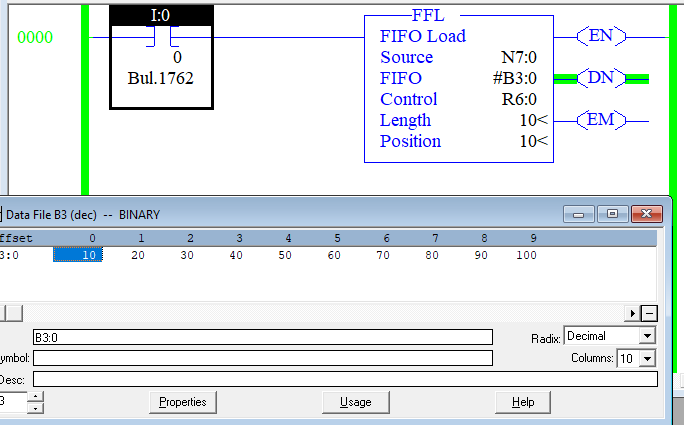So we have completed loading the values now the Position is at 10 and the done bit does to the true state.

Now we are going to look at the unloading of the data,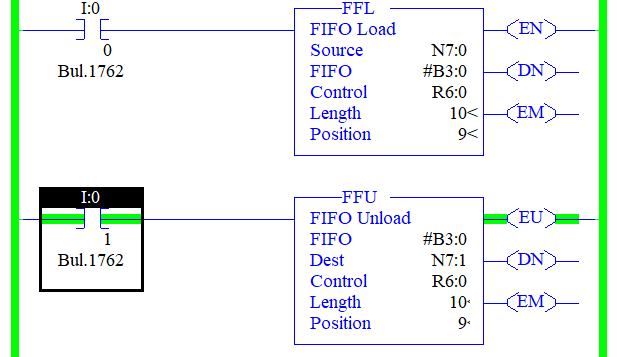Now I made the FFU rung true you can notice that the done bit goes OFF again.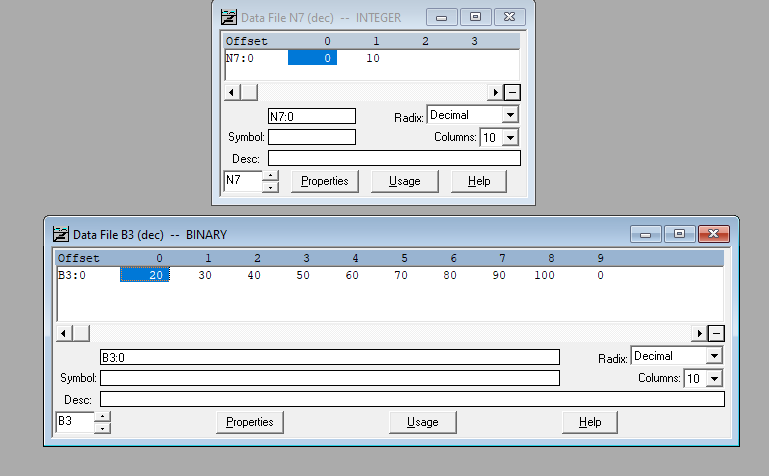Also, notice that the value which got loaded first comes out first. So the expansion of FIFO is called First in First Out. The one which came first unloads first.

Likewise whenever the rung makes false to true transition each value unloads in a manner that which came first goes out. So the last value which comes out should be 100.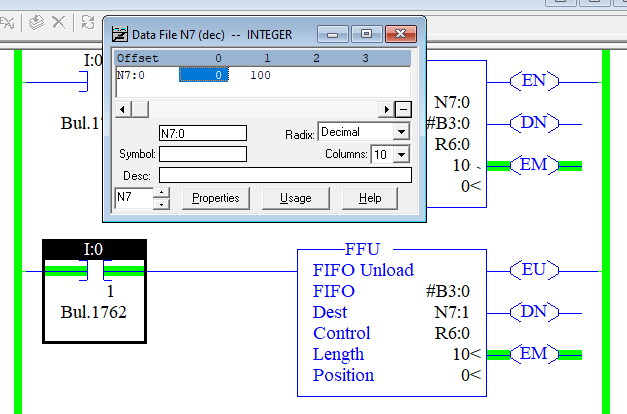You can also notice from the figure that the empty bit goes up when the values on the FIFO address become empty.

An example of a real-time application where FIFO is used might be tracking parts through an assembly line where parts are represented by values that have a part number and assembly code.